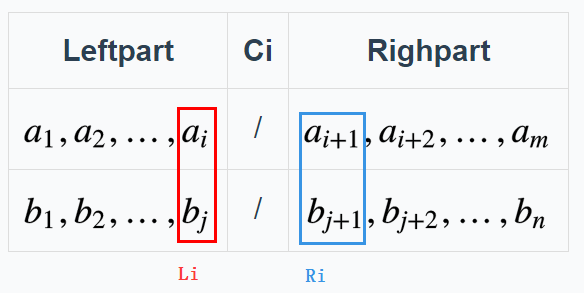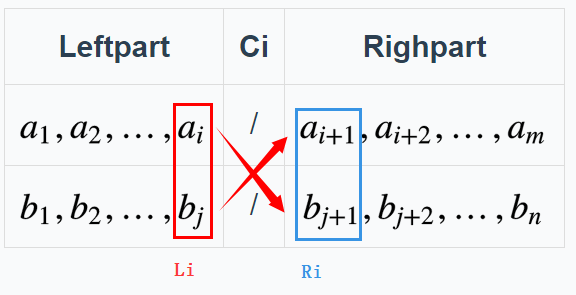# 【分步详解】两个有序数组中的中位数和Top K问题(这也是一道leetcode的经典题目：《LeetCode》解题笔记：004. Median of Two Sorted Arrays[H]

# 预备知识

## 先解释下“割”

Ps. 割可以割在两个数中间，也可以割在1个数上，如果割在一个数上，那么这个数即属于左边，也属于右边。（后面讲单数组中值问题的时候会说）

[2 3 / 5 7]

[2 3 (4/4) 5 7]

# 双数组

Ci $C_i$为第i个数组的割。
Li $L_i$为第i个数组割后的左元素.
Ri $R_i$为第i个数组割后的右元素。## 如何从双数组里取出第k个元素

1. 首先 Li<=Ri $L_i <= R_i$是肯定的（因为数组有序，左边肯定小于右边）
2. 如果我们让 L1<=R2 $L_1 <= R_2$ && L2<=R1 $L_2 <= R_1$1. 那么左半边 全小于右半边，如果左边的元素个数相加刚好等于k，那么第k个元素就是Max(L1,L2)，参考上面常识1。
2. 如果 L1>R2，说明数组1的左边元素太大（多），我们把C1减小，把C2增大。L2>R1同理，把C1增大，C2减小。

### 假设k=3

[1 4 7 9] $[1\ 4\ 7\ 9]$
[2 3 5] $[2\ 3\ 5]$

[1 4/7 9] $[1\ 4/ 7\ 9]$
[2/3 5] $[2/3\ 5]$

[1/4 7 9] $[1/4\ 7\ 9]$
[2 3/5] $[2\ 3/5]$

## 让数组恒为奇数

Ps.注意是虚拟加，其实根本没这一步，因为通过下面的转换，我们可以保证虚拟加后每个元素跟原来的元素一一对应

[1 4 7 9]4[# 1 # 4 # 7 # 9 #]9
[2 3 5]3[# 2 # 3 # 5 #]7

/原位置新位置除2后
0101
5252

## 在虚拟数组里表示“割”

Li = (Ci-1)/2
Ri = Ci/2

1. 割在4/7之间‘#’，C = 4，L=(4-1)/2=1 ，R=4/2=2

2. 割在3上，C = 3，L=(3-1)/2=1，R=3/2 =1，刚好都是3的位置！

Mid = (A[m+n+1]+A[m+n+2])/2
= (Max(L1+L2) + Min(R1+R2) )/2

# 分治的思路

## 怎么治？

- L1>R2，把C1减小，C2增大。—> C1向左二分
- L2>R1，把C1增大，C2减小。—> C1向右二分

## 越界问题

- C1 = 0 —— 数组1整体都比中值大，L1 >R2，中值在2中
- C2 = 0 —— 数组1整体都比中值小，L1

# 代码

    double findMedianSortedArrays(vector<int>& nums1, vector<int>& nums2) {
int n = nums1.size();
int m = nums2.size();
if(n > m)   //保证数组1一定最短
return findMedianSortedArrays(nums2,nums1);
int L1,L2,R1,R2,c1,c2,lo = 0, hi = 2*n;  //我们目前是虚拟加了'#'所以数组1是2*n长度
while(lo <= hi)   //二分
{
c1 = (lo+hi)/2;  //c1是二分的结果
c2 = m+n- c1;
L1 = (c1 == 0)?INT_MIN:nums1[(c1-1)/2];   //map to original element
R1 = (c1 == 2*n)?INT_MAX:nums1[c1/2];
L2 = (c2 == 0)?INT_MIN:nums2[(c2-1)/2];
R2 = (c2 == 2*m)?INT_MAX:nums2[c2/2];

if(L1 > R2)
hi = c1-1;
else if(L2 > R1)
lo = c1+1;
else
break;
}
return (max(L1,L2)+ min(R1,R2))/2.0;
}
};

06-20829005-271008
03-156476
09-292万+
11-091838
11-20649
03-0723
06-229347
01-26285
04-231748
10-25936
03-216791
05-201万+
02-1356
12-17231
10-1943
05-02133
05-31135
05-08231